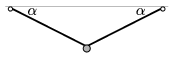Mathematical and Physical Journal
for High Schools
Issued by the MATFUND Foundation
 Already signed up? New to KöMaL?

#Problem P. 3926. (November 2006)

P. 3926. The two ends of a rubber string of negligible mass are fixed at the same height, such that the string is un-stretched. A small object is attached to the string in its midpoint, thus the angle of depression of the string is=30o. Then the small object is charged and vertical electric field is applied. Initially the depression angle of the string increases to1=45o, then the electric field is changed and the depression angle increases to2=60o. By what factor is the electric field in the second case more than that of in the first case?(4 pont)

Deadline expired on December 11, 2006.

### Statistics:

 146 students sent a solution. 4 points: 89 students. 3 points: 22 students. 2 points: 23 students. 1 point: 6 students. 0 point: 4 students. Unfair, not evaluated: 2 solutionss.

Problems in Physics of KöMaL, November 2006